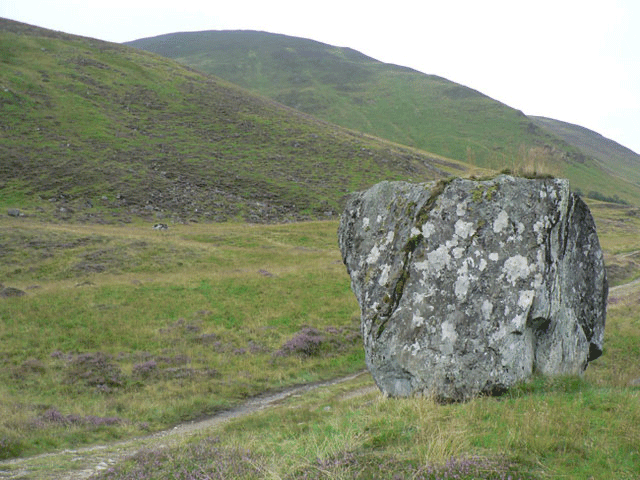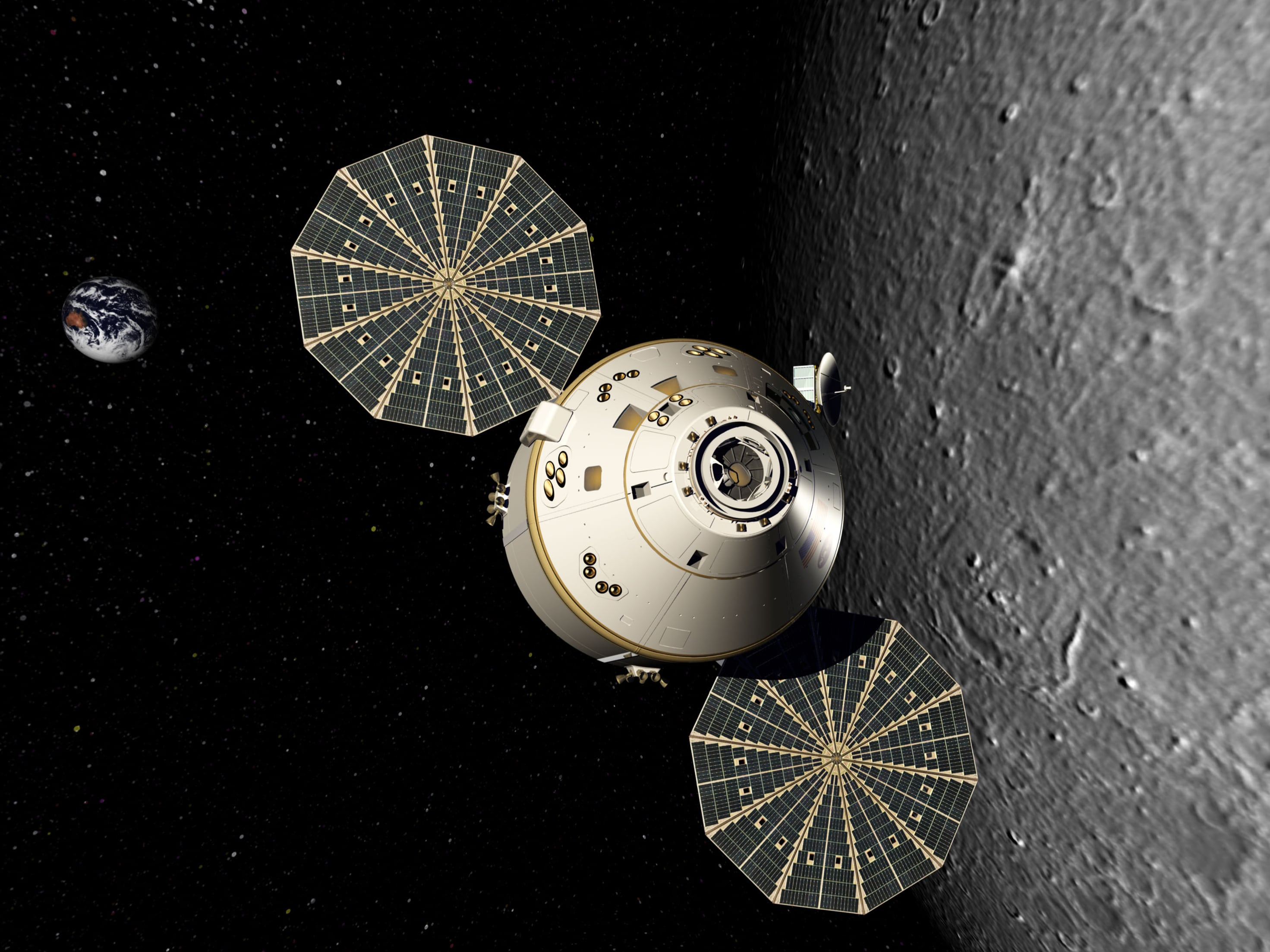# Engineering Statics: Open and Interactive

## Section1.1Newton’s Laws of Motion

The English scientist Sir Issac Newton established the foundation of mechanics in 1687 with his three laws of motion, which describe the relation between forces, objects and motion. Motion can be separated into two types:
• Translation— where a body changes position without changing its orientation in space, and
• Rotation— where a body spins about an axis fixed in space, without changing its average position.
Some moving bodies are purely translating, others are purely rotating, and many are doing both. Conveniently, we can usually separate translation and rotation and analyze them individually with independent equations.
Newton's three laws and their implications with respect to translation and rotation are described below.

### Subsection1.1.1Newton’s 1st Law

Newton’s first law states that
an object will remain at rest or in uniform motion in a straight line unless acted upon by an external force.
This law, also sometimes called the “law of inertia,” tells us that bodies maintain their current velocity unless a net force is applied to change it. In other words, an object at rest it will remain at rest and a moving object will hold its current speed and direction unless an unbalanced force causes a velocity change. Remember that velocity is a vector quantity that includes both speed and direction, so an unbalanced force may cause an object to speed up, slow down, or change direction.Figure 1.1.1. This rock is at rest with zero velocity and will remain at rest until a unbalanced force causes it to move.Figure 1.1.2. In deep space, where friction and gravitational forces are negligible, an object moves with constant velocity; near a celestial body gravitational attraction continuously changes its velocity.
Newton’s first law also applies to angular velocities, however instead of force, the relevant quantity which causes an object to rotate is called a torque by physicists, but usually called a moment by engineers. A moment, as you will learn in Chapter 4, is the rotational tendency of a force. Just as a force will cause a change in linear velocity, a moment will cause a change in angular velocity. This can be seen in things like tops, flywheels, stationary bikes, and other objects that spin on an axis when a moment is applied, but eventually stop because of the opposite moment produced by friction.
In the absence of friction this top would spin forever, but the small frictional moment exerted at the point of contact with the table will eventually bring it to a stop.

### Subsection1.1.2Newton’s 2nd Law

Newton’s second law is usually succinctly stated with the familiar equation
\begin{equation} \vec{F} = m \vec{a} \tag{1.1.1} \end{equation}
where $$\vec{F}$$ is net force, $$m$$ is mass, and $$\vec{a}$$ is acceleration.
You will notice that the force and the acceleration are in bold face. This means these are vector quantities, having both a magnitude and a direction. Mass on the other hand is a scalar quantity, which has only a magnitude. This equation indicates that a force will cause an object to accelerate in the direction of the net force, and the magnitude of the acceleration will be proportional to the net force but inversely proportional to the mass of the object.
In this course, Statics, we are only concerned with bodies which are not accelerating which simplifies things considerably. When an object is not accelerating $$a = 0\text{,}$$ which implies that it is either at rest or moving with a constant velocity. With this restriction Newton's Second Law for translation simplifies to
\begin{equation} \sum \vec{F}=0\tag{1.1.2} \end{equation}
where $$\sum \vec{F}$$ is used to indicate the net force acting on the object.
Newton’s second law for rotational motions is similar
\begin{equation} \vec{M} =I \boldsymbol{\alpha}\tag{1.1.3} \end{equation}
.
This equation states that a net moment $$\vec{M}$$ acting on an object will cause an angular acceleration $$\boldsymbol{\alpha}$$ proportional to the net moment and inversely proportional to $$I\text{,}$$ a quantity known as the mass moment of inertia. Mass moment of inertia for rotational acceleration is analogous to ordinary mass for linear acceleration. We will have more to say about the moment of inertia in Chapter 10.
Again, we see that the net moment and angular acceleration are vectors, quantities with magnitude and direction. The mass moment of inertia, on the other hand, is a scalar quantity and has only a magnitude. Also, since Statics deals only with objects which are not accelerating $$\boldsymbol{\alpha} = 0\text{,}$$ they will always be at rest or rotating with constant angular velocity. With this restriction Newton's second law implies that the net moment on all static objects is zero.
\begin{equation} \sum \vec{M}=0\tag{1.1.4} \end{equation}

### Subsection1.1.3Newton’s 3rd Law

Newton’s Third Law states
For every action, there is an equal and opposite reaction.
The actions and reactions Newton is referring to are forces. Forces occur whenever one object interacts with another, either directly like a push or pull, or indirectly like magnetic or gravitational attraction. Any force acting on one body is always paired with another equal-and-opposite force acting on some other body.Figure 1.1.4. The earth exerts a gravitational force on the moon, and the moon exerts an equal and opposite force on the earth.
These equal-and-opposite pairs can be confusing, particularly when there are multiple interacting bodies. To clarify, we always begin solving statics problems by drawing a free-body diagram — a sketch where we isolate a body or system of interest and identify the forces acting on it, while ignoring any forces exerted by it on interacting bodies.
Consider the situation in figure Figure 1.1.5. Diagram (a) shows a book resting on a table supported by the floor. The weights of the book and table are placed at their centers of gravity. To solve for the forces on the legs of the table, we use the free-body diagram in (b) which treats the book and the table as a single system and replaces the floor with the forces of the floor on the table. In diagram (c) the book and table are treated as independent objects. By separating them, the equal-and-opposite interaction forces of the book on the table and the table on the book are exposed.